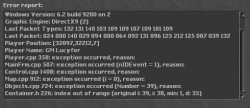# C++TFS 1.2 Non stop debugs

#### Roni123

##### Hardstyle Never Die < 3 !!!
Hello,

Dears,

I have problem with debugs but I don't know when they appear I have detected them in POI but later I have create monster in city and the same, when I make e.g 1 demon and 1 lich going to nothing but when I make Demon and Undead Dragon, then after 3-4 secound when they goin to attack I got debugs,BTW.
Its a 8.0 Distro.

Update
Ok I think there are problem with monster Undead Dragon he maked something on my character what debug him because when got debug I have restarted server and after restart I'm login on character and after 1 second got debug till to character die maybe someone can check this monster lua

Lua:
``````<?xml version="1.0" encoding="UTF-8"?>
<health now="8350" max="8350"/>
<look type="231" corpse="6306"/>
<targetchange interval="4000" chance="10"/>
<flags>
<flag summonable="0"/>
<flag attackable="1"/>
<flag hostile="1"/>
<flag illusionable="1"/>
<flag convinceable="0"/>
<flag pushable="0"/>
<flag canpushitems="1"/>
<flag canpushcreatures="1"/>
<flag targetdistance="1"/>
<flag staticattack="70"/>
<flag runonhealth="300"/>
</flags>
<attacks>
<attack name="melee" interval="2000" skill="90" attack="96"/>
<attack name="physical" interval="2000" chance="5" range="7" radius="4" target="1" min="-300" max="-400">
<attribute key="areaEffect" value="redspark"/>
</attack>
<attack name="physical" interval="2000" chance="10" range="7" min="-125" max="-600">
<attribute key="shootEffect" value="death"/>
<attribute key="areaEffect" value="mortarea"/>
</attack>
<attack name="earth" interval="2000" chance="5" range="7" radius="4" target="1" min="-100" max="-390">
<attribute key="shootEffect" value="poison"/>
<attribute key="areaEffect" value="poison"/>
</attack>
<attack name="earth" interval="2000" chance="15" target="1" range="7" min="-0" max="-180">
<attribute key="shootEffect" value="poison"/>
<attribute key="areaEffect" value="poison"/>
</attack>
<attack name="earth" interval="2000" chance="10" length="8" spread="3" min="-150" max="-690">
<attribute key="areaEffect" value="poison"/>
</attack>
<attack name="lifedrain" interval="2000" chance="10" length="8" spread="3" min="-300" max="-700">
<attribute key="areaEffect" value="redshimmer"/>
</attack>
<attack name="lifedrain" interval="2000" chance="10" radius="3" min="-100" max="-200">
<attribute key="areaEffect" value="redshimmer"/>
</attack>
<attack name="undead dragon curse" interval="2000" chance="10"/>
</attacks>
<defenses armor="40" defense="40">
<defense name="healing" interval="2000" chance="15" min="200" max="250">
<attribute key="areaEffect" value="blueshimmer"/>
</defense>
</defenses>

<immunities>
<immunity fire="1"/>
<immunity earth="1"/>

<immunity paralyze="1"/>
<immunity invisible="1"/>
<immunity drown="1"/>
</immunities>
<voices interval="5000" chance="10">
<voice sentence="FEEEED MY ETERNAL HUNGER!" yell="1"/>
<voice sentence="I SENSE LIFE" yell="1"/>
</voices>
<loot>
<item id="2148" countmax="100" chance="35500"/><!-- gold coin -->
<item id="2148" countmax="98" chance="55500"/><!-- gold coin -->
<item id="6500" chance="12460"/><!-- demonic essence -->
<item id="2152" countmax="5" chance="52000"/><!-- platinum coin -->
<item id="6300" chance="1150"/><!-- death ring -->
<item id="2033" chance="6002"/><!-- golden mug -->
<item id="2547" countmax="15" chance="15190"/><!-- power bolt -->
<item id="7430" chance="4000"/><!-- dragonbone staff -->
<item id="2476" chance="5500"/><!-- knight armor -->
<item id="2177" chance="2500"/><!-- life crystal -->
<item id="5925" chance="14180"/><!-- hardened bone -->
<item id="2498" chance="1720"/><!-- royal helmet -->
<item id="2454" chance="1290"/><!-- war axe -->
<item id="2146" countmax="2" chance="28370"/><!-- small sapphires -->
<item id="7368" countmax="5" chance="26650"/><!-- assassin stars -->
<item id="7402" chance="860"/><!-- dragon slayer -->
<item id="2144" countmax="2" chance="22780"/><!-- black pearls -->
<item id="2466" chance="860"/><!-- golden armor -->
</loot>
</monster>``````

Can Close ! There are problem with lifedrain of this monster what wasn't at 8.0.

Thanks.

Last edited: